## 2. 5. 6 Accumulation in Depth

In the following section a summation is considered in which different traversed elements are required in the summand function in order to evaluate quantities. A quantity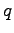has to be evaluated in a traversed vertexwhereas a quantity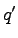is evaluated in the base element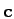of the traversal. The non-functional description of the summation can be written as follows: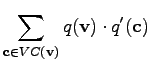(2.50)

Using the methods from Section 2.5.3 the expression can not be transformed into a function that can be written as unary function to be evaluated in the vertex. The main reason for this problem is that only the cellis passed to the inner function, whereas the vertexis not available and, therefore, can not be passed to the summand function.

A simple method with which the argument value of the outside argument can be preserved when changing the argument list is based on named variables. Before the summation is carried out, a named variable, in this case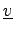is assigned the (vertex) value of the argument passed. When the summands are evaluated, the function argument is the traversed cell incident to the initial vertex. However, the named variablecan be accessed in analogy to an unnamed variable. A formulation of (2.51) using only functional expressions and named variables is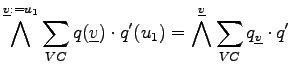(2.51)

The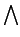operator is used in order to explicitly assign the named variablethe value that is currently provided in the function argument. In analogy to unnamed functions, named functions can be written as underlined indices when quantities are applied on them. Furthermore, the definition of the named variable as the first argument (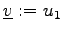) can be simplified, because it is generally assumed that the first argument is preserved in order not to be overwritten or discarded in the summation. Therefore, the assignment can be neglected and only the name is written. Furthermore, the convention is introduced that named variables are underlined in order to distinguish them from other terms.

Compared to the lambda function which is known from the lambda calculus [67,68], the order of the function remains unchanged, whereas named variables obtain a certain value. An appropriate formulation can also be obtained by the lambda function, where the use of named variables can be avoided. For the sake of clarity and conciseness it turned out to be more appropriate to use this formulation.

The summation can be further simplified by collapsing the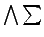formalism to a common summation symbol. The notation can be written shorter, without loss of generality. This reasoning can of course be applied to all accumulation mechanisms.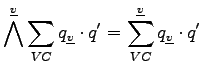(2.52)

Using schemes of this type, arbitrary formulae which especially contain summations with function evaluation on different topological entities can be obtained. For simple equations, the formalisms can be specified easily and no specification overhead is required. For more complicated expressions a concise and explicit manner of specification is provided.

Michael 2008-01-16